Mathematics
Easy

Question

# Which property listed is the reason for the last step in the proof?GIVEN: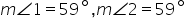PROVE: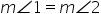Statements Reasons 1.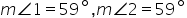1. Given 2.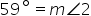2. Symmetric Property of Equality 3.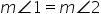3. _____?

## Transitive Property of EqualityReflexive Property of EqualitySymmetric Property of EqualityDistributive PropertyHint:

## The correct answer is: Transitive Property of Equality

### Here we have to find what is property of m∠1=m∠2.Firstly, we have given that m∠1=59° and m∠2=59°. And 59°= m∠1 and this property is symmetric.In symmetric , if A = B then B = A as well.So m∠1=59° and 59°= m∠1 then we can also write m∠2=59° then 59°= m∠2Here, m∠1=59° and m∠2=59° and also m∠1=m∠2. Lets here A = m∠1 and B = 59° and C = m∠2Here , m∠1=59° [A = B] And, 59°= m∠2 [B = C]Note, in transition, A =B and B = C then A =C ,There fore, we can write , m∠1=m∠2 . So the property is Transitive property of Equality.The correct answer is Transitive property of Equality.

Here we have,  find the property of m∠1=m∠2 which is transitive. In transitive if A = B and B = C is given then it is also A = C .

### Related Questions to study#### With Turito Foundation.#### Get an Expert Advice From Turito.